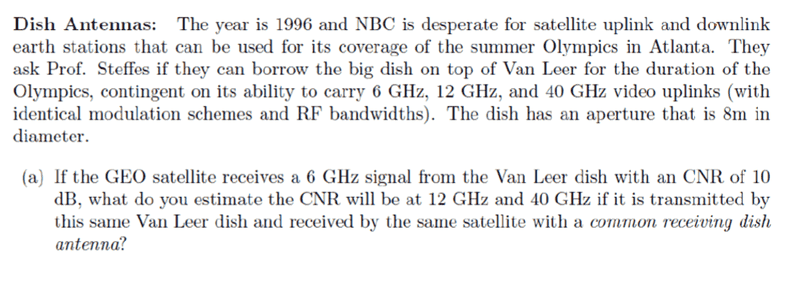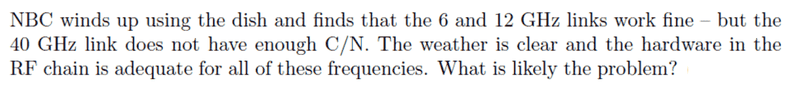# Dish Antennas C/N Problem

ashah99
Thread moved from the technical forums to the schoolwork forums
Summary:: I am stuck on a problem on dish antenna C/N and it's relation to frequency. Please see below for thought process.

Problem Statement:,
Link budget formula in dB form: Pr = Pt + Gt + Gr + 20*log10(lambda/(4*pi*distance)) - Losses [dB]
C/N = Pr - Pn [dB], where Pn = 10*log10(k*T*B)

I am stuck on coming up with the final answer. Without knowing all the details (RX/TX powers, antenna efficiency, additional losses, etc.), I can only conclude that some factors are equal, like noise power. The antenna gains Gt, Gr, and free space propagation losses all are frequency dependent and are proportional to f^2, so with 2 gain factors and 1 loss, my net C/N increase would be with respoect to 1 f^2. I'm just not sure how to proceed from here. any help is very much appreciated.

Gold Member
Antenna gain ∝ 10 log (pi D / lambda)^2
So gain rises with freq squared.
At 12 GHz:-
Increase in gain of Gt at 12 GHz = 20 log 12/6 = 6dB
Increase in gain of Gr at 12 GHz = 6dB
Path loss is also dependent on freq squared, so it will increase by 6dB at 12 GHz
Pr change = Gt + Gr - Lp = 6 + 6 - 6 = +6dB
CNR = 10 + 6 = 16dB
At 40GHz:-
Increase in Gt at 40 GHz = 20 log 40/6 = 16,5dB
Increase in gain of Gr = 16.5 dB
Path loss increase = 20 log 40/6 = 16.5 dB
Pr change = Gt+Gr -Lp = 16.5 + 16.5 - 16.5 = +16.5 dB
CNR = 10 + 16.5 = 26.5 dB

ashah99
Antenna gain ∝ 10 log (pi D / lambda)^2
So gain rises with freq squared.
At 12 GHz:-
Increase in gain of Gt at 12 GHz = 20 log 12/6 = 6dB
Increase in gain of Gr at 12 GHz = 6dB
Path loss is also dependent on freq squared, so it will increase by 6dB at 12 GHz
Pr change = Gt + Gr - Lp = 6 + 6 - 6 = +6dB
CNR = 10 + 6 = 16dB
At 40GHz:-
Increase in Gt at 40 GHz = 20 log 40/6 = 16,5dB
Increase in gain of Gr = 16.5 dB
Path loss increase = 20 log 40/6 = 16.5 dB
Pr change = Gt+Gr -Lp = 16.5 + 16.5 - 16.5 = +16.5 dB
CNR = 10 + 16.5 = 26.5 dB

Hi tech99. Thank you for your reply and help. I'm a little confused on how you went
from
G_ant = 10 log (pi D / lambda)^2 or 20 log (pi D / lambda) = 20 log (pi (8 m) / [(3e8 m/s)/ 12e9 Hz)] ) to
20 log 12/6 above for the Increase in gain of Gt/Gr at 12 GHz.
Would you be willing to explain?

Gold Member
If we take the log of a number squared, that is the same as twice the log of the number not squared.
10 log N^2 = 20 log N.
I think that is the confusion.

ashah99
If we take the log of a number squared, that is the same as twice the log of the number not squared.
10 log N^2 = 20 log N.
I think that is the confusion.
Not really. I understand that. I’m having trouble understanding how you got the 20log(12/6) and 20log(40/6) parts above.

Gold Member
The antenna power gain is proportional to frequency squared. If we increase the frequency from 6 to 12 GHz, the power gain rises by (12/6)^2 = 4. Or in decibal form Gain Ratio = 20 log 12/6.
The 40/6 mentioned is the frequency ratio between 6 GHz and 40 GHz.

ashah99
The antenna power gain is proportional to frequency squared. If we increase the frequency from 6 to 12 GHz, the power gain rises by (12/6)^2 = 4. Or in decibal form Gain Ratio = 20 log 12/6.
The 40/6 mentioned is the frequency ratio between 6 GHz and 40 GHz.

I see that now. Thanks for the explanations; this cleared things up. Would you mind if I annoyed you with a follow-up question to this scenerio?As to why this antenna will fail to deliver more power gain at the higher frequency, I can only think of atmospheric attentuation, mostly rain, but the problem says that the weather is clear. I'm not an expert at antenna design, do you have any thoughts?